## Chemical Equations:

Chemical equations are the symbolic representation of chemical reactions where the reactants and products are written in the form of symbols and formulae on the Left hand side and right hand side of an arrow respectively.
Example of a chemical equation:
CaCl2 + 2AgNO3 → Ca(NO3)2 + 2AgCl
In the above equation, the reactants calcium chloride and silver nitrate react to form the products calcium nitrate and silver chloride.

## Word Equations:

One way of representing chemicals reactions is word equation which is a short and simplest way of writing chemical reactions as compared to the description of a chemical reaction in a sentence form which is quite long.
The word equation for the reaction between magnesium and oxygen gas would be:## Representation of Chemical Reactions:

To make chemical equations more concise and useful we use chemical formulae instead of words. A chemical equation represents a chemical reaction. The word equation shown above can be written as:
Mg + O2 → MgO## Balanced and Unbalanced Chemical Equations:

A balanced chemical equation has an equal number of atoms of different elements in the reactants and products in accordance with the law of conservation of mass.

Number of atoms of each element in reactant side = Number of atoms of each element in product side
An unbalanced chemical equation has an unequal number of atoms of one or more elements in the reactants and products because the mass is not the same on both sides of the equation. Such a chemical equation is a skeletal chemical equation for a reaction. The equation
Mg + O2 → MgO
is a skeletal chemical equation as the number of atoms of oxygen is not equal in reactant side (2 atoms) and product side (1 atom).

## Balanced Chemical Equations:

We need to balance chemical equations to satisfy the law of conservation of mass in chemical reactions. The process of making the number of different types of atoms equal on both the sides of an equation is called balancing of equation.

Hit-and-trial Method of Balancing Chemical Equations
Consider the chemical equation for the reaction between methane and oxygen to yield carbon dioxide and water:
CH4 + O2 → CO2 + H2O
The above equation is an unbalanced equation as the number of atoms of both hydrogen and oxygen are not equal on reactant side and product side.
Step 1: First draw boxes around each formula or put a bracket around each formula. Do not change anything inside the boxes or brackets while balancing the equation.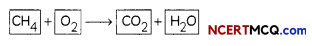Step II: List the number of atoms of different elements present in the unbalanced equationStep III: Start balancing with the compound that contains the maximum number of atoms. It may be a reactant or a product. In that compound, select the element which has the maximum number of atoms.

Here, we select CH4 and the element hydrogen in it.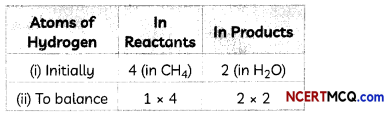To make the number of atoms of hydrogen equal, we put the coefficient ‘2’ as ‘2H2O’

→ To equalize the number of atoms, we cannot alter the formulae of the compounds or elements involved in the reactions.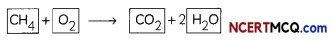This is a partly balanced equation as the number of oxygen atoms are not balanced.

Step IV: Pick any of those elements whose atoms are still not balanced to proceed further.
As number of oxygen atoms are not equal on reactant and product sides, we will balance O atoms now.Step V: Count atoms of each element on both sides of the equation to check the correctness of the balanced equation.
CH4 + 2O2 → CO2 + 2H2O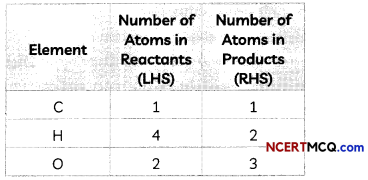The numbers of atoms of elements on both sides of above equation are equal. This equation is now balanced.

Step VI: Writing Symbols of Physical States:
To make a chemical equation more informative, the physical states of the reactants and products are mentioned along with their chemical formulae. For example, the burning of methane in oxygen can be written as:
CH4(g) + 2O2(g) → CO2(g) + 2H2O(g) + HeatExample 1.
Balance the chemical equation Ca + H2O → Ca(OH)2 + H2.
Steps to balance the equation are as follows:
Step I: First draw boxes around each formula or put a bracket around each formula.
Ca + H2O → Ca(OH)2 + H2

Step II: List the number of atoms of different elements present in the unbalanced equation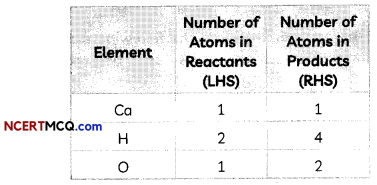We note here that both H and O atoms are not equal on both sides.

Step III: We first equalize atoms of H.To make the number of atoms of hydrogen equal, we put the coefficient ‘2’ as 2H20
Ca + 2H2O → Ca(OH)2 + H2
By putting the coefficient 2 before H2O, the atoms of both H and O get multiplied by 2.

We now count atoms of each element on both sides of the equation to check the correctness of the equation.This is a balanced equation as the number of hydrogen and oxygen atoms are now balanced.

So, the balanced equation is:
Ca + 2H2O → Ca(OH)2 + H2

Example 2.
Balance the following chemical equations.
(A) HNO3 + Ca(OH)2 → Ca(NO3)2 + H2O
2HNO3 + 2Ca(OH)2 → Ca(NO3)2 + 2H2O

(B) NaOH + H2SO4 → Na2SO4 + H2O
2NaOH + 3H2SO4 → Na2SO4 + 2H2O

(C) NaCl + AgNO3 → AgCl + NaNO3
NaCl + AgNO3 → AgCl+NaNO3

(D) BaCl2 + H2SO4 → BaSO4 + HCl
BaCl2 + H2SO4 → BaSO4 + 2HClExample 3.
Write the balanced chemical equations for the following reactions.
(A) Calcium hydroxide + Carbon dioxide → Calcium carbonate + Water
Ca(OH)2 + CO2 → CaCO3 + H2O

(B) Zinc + Silver nitrate → Zinc nitrate + Silver
Zn + 2AgNO → Zn(NO3)2 + 2Ag

(C) Aluminium + Copper chloride → Aluminium chloride + Copper
2Al + 3CuCl2 → 2AlCl3 + 3Cu

(D) Barium chloride + Potassium sulphate → Barium sulphate + Potassium chloride
BaCl2 + K2SO4 → BaSO4 + 2KCl

Caution
Students should not alter the formulae of compounds or elements involved in the reactions to equalize the number of atoms while balancing a chemical equation.

For example, for balancing the equation
AlCl3(aq) + NH4OH(oq) → Al(OH)3(s) + NH4Cl(aq)

we observe that number of Chlorine atoms am not equal on both sides. But we cannot balance the equation by writing NH4Cl3 in place of NH4Cl as in
AlCl3(aq) + NH4OH(aq) → Al(OH)3(s) + NH4Cl3(aq).

Instead, we have to balance as:
AlCl3(aq) + 3NH4OH(aq) → Al(OH)3(s) + 3NH4Cl(aq)

We should write the formulae of each compound by using their correct symbols and valencies. If the formula of even a single compound is incorrect, the entire equation will be incorrect.

For example, for writing a balanced equation for the reaction: when copper oxide is heated with magnesium powder, magnesium oxide and copper are formed as magnesium displaces copper from its oxide, if we write formula of copper oxide as CuO2 or Cu20 instead of CuO, the equation will be incorrect.

The correct equation is:
Cu(s) + Mg(s) → MgO(s) + Cu(s)

In order to write and balance the equation correctly, it is important to remember the seven elements that exist in nature as diatomic molecules (H2, N2, O2, F2, Cl2, Br2, and I2)

Whenever word equations are given along with the physical states of reactants and products, the same should also be written in the chemical equations.

Indicate the Physical States of Reactants and Products in an Equation

1. The Solid-state is indicated by the symbol (s). Formation of precipitate is indicated by writing (s) or downward pointing arrow (l)
2. Liquid state is indicated by the symbol (l)
3. Aqueous state (solution made in water) is indicated by the symbol (aq)
4. Gaseous state is indicated by the symbol (g) or an upward pointing arrow (t).

For example, in the chemical equation
Fe(S) + CuSCO4(aq) → FeSO4(aq)+ Cu

Fe is in solid-state, CuSO4 is in the aqueous state, FeSO4 is in an aqueous state and Cu is in solid state.

Indicate the Heat Changes in an Equation:

1. An exothermic reaction (reaction in which heat is evolved) is indicated by writing “+ Heat” on the products side of an equation.
2. An endothermic reaction (reaction in which heat is absorbed) is indicated by writing “+ Heat” on the reactants side of an equation.

Indicate the Conditions under which the Reaction Takes Place
The reaction conditions, such as temperature, pressure, catalyst, etc., for the reaction may be indicated above and/or below the arrow in the equation.

For example, the temperature at which sodium hydrogen carbonate is heated to obtain sodium carbonate, carbon dioxide and water can be represented by the chemical equation,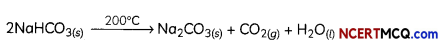Example 4.
Write a balanced chemical equation with state symbols for the following reactions.
(A) Solutions of barium chloride and sodium sulphate in water react to give insoluble barium sulphate and the solution of sodium chloride.
BaCl2(aq) + Na2SO4(aq) → BaSO4(s) + 2NaCl (aq)

(B) Sodium hydroxide solution (in water) reacts with a hydrochloric acid solution (in water) to produce sodium chloride solution and water.
NaOH(aq)) + HCl(aq) → NaCL(oq) + H2O(l)

Example 5.
Translate the following statements into chemical equations and balance them.
(A) Hydrogen gas combines with nitrogen to form ammonia.
3H2(g) + N2(g) → 2NH3(g)

(B) Hydrogen sulphide gas burns in air to give water and sulphur dioxide.